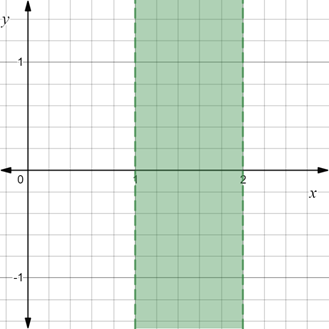# To graph : The region provided by the set { ( x , y ) | 1 &lt; x &lt; 2 } .### Precalculus: Mathematics for Calcu...

6th Edition
Stewart + 5 others
Publisher: Cengage Learning
ISBN: 9780840068071### Precalculus: Mathematics for Calcu...

6th Edition
Stewart + 5 others
Publisher: Cengage Learning
ISBN: 9780840068071

#### Solutions

Chapter 1.8, Problem 27E
To determine

## To graph: The region provided by the set {(x,y)|1<x<2} .

Expert Solution

### Explanation of Solution

Given information:

The set, {(x,y)|1<x<2} .

Graph:

The graph of the region provided by the set {(x,y)|1<x<2} is sketched in the coordinate plane.

Construct broken vertical lines x=1 and x=2 and shaded the region between it.

The region obtained is provided below,Interpretation:

The region provided by the set {(x,y)|1<x<2} is shaded region. The vertical line at x=1 and x=2 are broken lines because they are not included in the set.The region consists of all those points whose x-coordinate lie between x=1 and x=2 . Therefore, shaded region is the region between vertical lines x=1 and x=2 and these two vertical lines are not included in the region.

### Have a homework question?

Subscribe to bartleby learn! Ask subject matter experts 30 homework questions each month. Plus, you’ll have access to millions of step-by-step textbook answers!